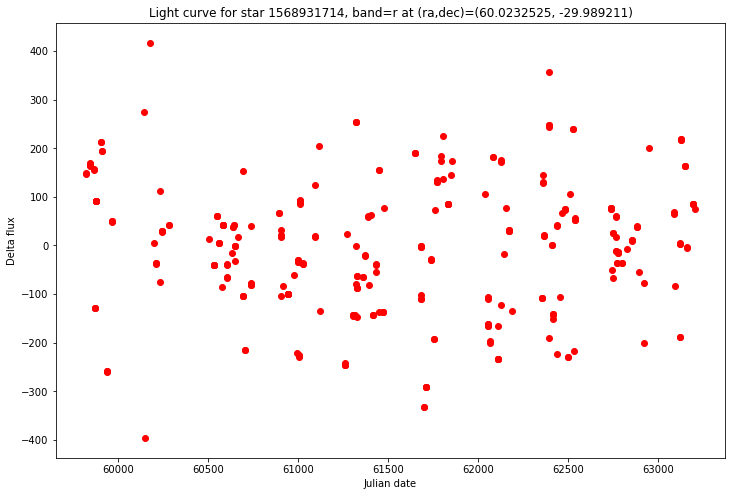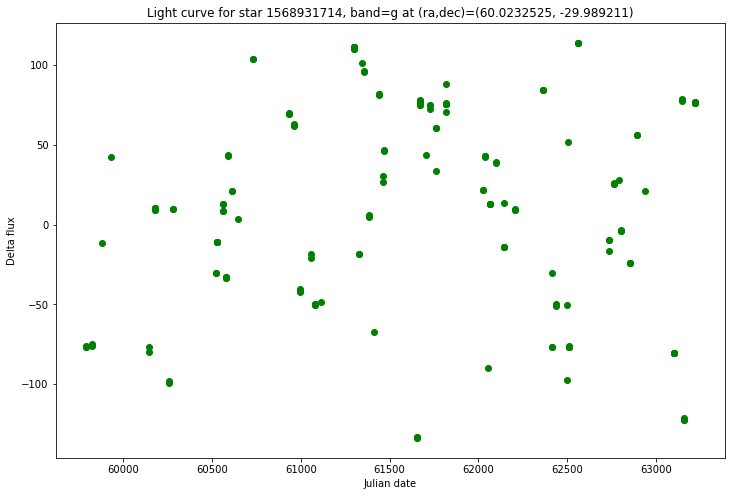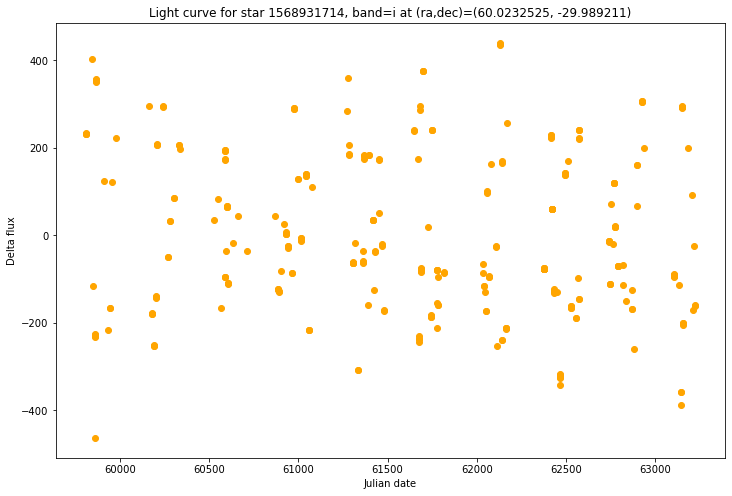# Accessing DC2 truth and simulated observations data in PostgreSQL at NERSC¶

Owner: Joanne Bogart @jrbogart
Last Verified to Run: 2020-11-24

This notebook demonstrates access to truth catalog data via the PostgreSQL database at NERSC. Currently (May 1, 2020) two kinds of truth catalogs are available: star truth and supernova truth. This notebook will concentrate on star truth. The minion observation database is also available, as well as the Run2.2i dr6 object catalog (dpdd columns approximating specification in LSE-163 only).

Learning objectives:

After going through this notebook, you should be able to:

1. Find out what star truth and simulated observation information is available and query it.
2. Make use of standard tools to, e.g., plot light curves

Logistics: This notebook is intended to be run through the JupyterHub NERSC interface available here: https://jupyter.nersc.gov. To setup your NERSC environment, please follow the instructions available here: https://confluence.slac.stanford.edu/display/LSSTDESC/Using+Jupyter+at+NERSC

### Conventions¶

• SQL keywords have been written in ALL CAPS only to make them stand out in queries. (The database server ignores case in queries for keywords, column names and table names.)
In :
import psycopg2
import numpy as np
%matplotlib inline
import matplotlib.pyplot as plt
import pandas as pd


Make the db connection

In :
dbname = 'desc_dc2_drp'
dbuser = 'desc_dc2_drp_user'
dbhost = 'nerscdb03.nersc.gov'
dbconfig = {'dbname' : dbname, 'user' : dbuser, 'host' : dbhost}
dbconn = psycopg2.connect(**dbconfig)

In :
obs_schema = 'minion_test'
truth_schema = 'star_truth'
object_schema = 'run22i_dr6_wfd_v1'
#truth_schema = 'sne_truth'


Initial steps in this notebook can be run for supernova truth instead of star truth just by changing the value for truth_schema above, but the section labeled Sample Query and the light curve queries need more adjustments.

Convenience utilities, defined here so as not to clutter up the main line.

In :
def get_schema_tables(conn, schema):
'''
Returns 1-d numpy array of table names
'''
q = f"""SELECT DISTINCT table_name FROM information_schema.columns
WHERE table_schema='{schema}'
ORDER BY table_name"""
with conn.cursor() as cursor:
cursor.execute(q)
records = cursor.fetchall()
tables = np.array([r for r in records])
return tables

In :
def get_table_columns(conn, schema, table):
'''
Returns a pandas dataframe with columns column_name, datatype
'''
q = f"""SELECT column_name, data_type FROM information_schema.columns
WHERE table_schema='{schema}' AND table_name='{table}'
ORDER BY column_name"""
with conn.cursor() as cursor:
cursor.execute(q)
records = cursor.fetchall()
print(len(records))
df = pd.DataFrame(records, columns=['column_name', 'data_type'])
return df



Another convenience routine. It makes use of the public function conesearch and provides a place to document its arguments. See also a more comprehensive list of such functions.

In :
def format_cone_search(coord_column, ra, dec, radius):
'''
Parameters
coord_column:  name of column of type earth in the table
ra:  ra value at center of cone (degrees)
dec:  dec value at center of cone (degrees)

Returns
Condition to be inserted into WHERE clause for the query
'''
return cond


Display all tables belonging to the schema.

In :
schemas = [truth_schema, obs_schema]
for s in schemas:
tables = get_schema_tables(dbconn, s)
print(f"\nTables for schema {s}:")
for t in tables:
print(t)

Tables for schema star_truth:
stellar_variability_stats
stellar_variability_truth
truth_summary

Tables for schema minion_test:
cloud
config
config_file
field
log
missedhistory
obshistory
obshistory_proposal
proposal
proposal_field
seeing
seqhistory
seqhistory_missedhistory
seqhistory_obshistory
session
slewactivities
slewhistory
slewmaxspeeds
slewstate
summary
timehistory


Display all columns belonging to a couple tables

In :
truth_summary_info = get_table_columns(dbconn, truth_schema, 'truth_summary' )
truth_summary_info.style.set_properties(**{'text-align': 'left'})
truth_summary_info

20

Out:
column_name data_type
0 coord USER-DEFINED
1 dec double precision
2 flux_g real
3 flux_g_nomw real
4 flux_i real
5 flux_i_nomw real
6 flux_r real
7 flux_r_nomw real
8 flux_u real
9 flux_u_nomw real
10 flux_y real
11 flux_y_nomw real
12 flux_z real
13 flux_z_nomw real
14 host_galaxy bigint
15 id text
16 is_pointsource integer
17 is_variable integer
18 ra double precision
19 redshift real
In :
stellar_variability_info = get_table_columns(dbconn, truth_schema, 'stellar_variability_truth' )
stellar_variability_info.style.set_properties(**{'text-align': 'left'})
stellar_variability_info

5

Out:
column_name data_type
0 bandpass text
1 delta_flux double precision
2 id text
3 mjd double precision
4 obshistid integer

The most generally useful table in the obs database is summary. Note ra and dec are stored in radians in this table.

In :
obs_summary_info = get_table_columns(dbconn, obs_schema, 'summary' )
obs_summary_info.style.set_properties(subset=["column_name", "data_type"], **{'text-align': 'right'})
obs_summary_info

54

Out:
column_name data_type
0 airmass real
1 altitude double precision
2 azimuth double precision
3 darkbright real
4 descditheredcoord USER-DEFINED
5 descdithereddec double precision
6 descditheredra double precision
7 descditheredrottelpos double precision
8 dist2moon double precision
9 ditheredcoord USER-DEFINED
10 dithereddec double precision
11 ditheredra double precision
12 expdate integer
13 expmjd double precision
14 fieldcoord USER-DEFINED
15 fielddec real
16 fieldid integer
17 fieldra real
18 filter text
19 filtskybrightness real
20 finrank real
22 fwhmeff real
23 fwhmgeom real
24 humidity real
25 lst double precision
26 miescatter real
27 moonalt double precision
28 moonaz double precision
29 moonbright real
30 mooncoord USER-DEFINED
31 moondec double precision
32 moonillum real
33 moonphase real
34 moonra double precision
35 night integer
36 obshistid integer
37 phaseangle double precision
38 propid integer
39 rawseeing real
40 rotskypos double precision
41 rottelpos double precision
42 rscatter real
43 sessionid integer
44 slewdist real
45 slewtime double precision
46 solarelong double precision
47 sunalt double precision
48 sunaz double precision
49 transparency real
50 visitexptime double precision
51 visittime double precision
52 vskybright real
53 wind real

### Sample Query¶

Find delta flux readings for stars which are expected to be in the field of view for a particular visit. This sort of query returns practically instantly. In the stellar_variability_truth both of the columns mentioned in the WHERE clause - id and obshistid - are indexed.

In :
obshistid = 731791
table = 'stellar_variability_truth'
ids = ["'835183'", "'31303590103'","'31102013522'","'31303588649'", "'30317268917'",
"'30825472052'","'835279'","'31102039372'","'30825477672'","'31102046245'",
"'30321363109'","'31102051190'","'31102061342'","'30321363877'","'31102061079'",
"'31411663457'", "'31107813412'"]
id_list = ",".join(ids)

flux_q = f"""SELECT id, delta_flux FROM {truth_schema}.{table}
WHERE (obshistid={obshistid})
AND id IN ({id_list});"""
print(flux_q)
with dbconn.cursor() as cursor:
cursor.execute(flux_q)
f_records = cursor.fetchall()
df_flux = pd.DataFrame(f_records, columns=['id', 'delta_flux'])
df_flux

SELECT id, delta_flux FROM star_truth.stellar_variability_truth
WHERE (obshistid=731791)
AND id IN ('835183','31303590103','31102013522','31303588649','30317268917','30825472052','835279','31102039372','30825477672','31102046245','30321363109','31102051190','31102061342','30321363877','31102061079','31411663457','31107813412');

Out:
id delta_flux
0 30825477672 -30241.496100
1 31102061079 -24522.487526
2 31102039372 -2895.587106
3 31303590103 11601.473802
4 31102013522 47050.033179
5 31107813412 -21383.346704
6 31102061342 -10998.545541
7 30321363109 39996.367988
8 31102051190 -34863.941218
9 30317268917 81.519569
10 31303588649 -51343.699130
11 835279 73170.282195
12 835183 145258.546118

### Area searches¶

The column coord in table truth_summary and columns with "coord" in their names in the observation summary table are of a special type earth. The value is a triple of double precision numbers describing the position on a unit sphere corresponding to ra and dec. Indexes have been defined on these columns to speed up area searches.

The observation with obshistid=14 has desc dithered ra, dec = (1.69831745204, -0.59856) in radians. Find all observations within a radius (expressed in arcseconds).

Warning: All ra, dec in the observation summary table are in radians. ra and dec in truth_summary are in degrees. format_cone_search needs ra, dec in degrees.

In :
radius = 500
ra_deg = np.degrees((1.69831745204,))
dec_deg = np.degrees((-0.59856,))
dec = -0.59856
cond = format_cone_search('descditheredcoord', ra_deg, dec_deg, radius)
obs_query = f"""SELECT obshistid,descditheredra,descdithereddec FROM {obs_schema}.summary
WHERE {cond}"""
# Uncomment the following to confirm that the query looks reasonable
#print(obs_query)
with dbconn.cursor() as cursor:
%time cursor.execute(obs_query)
records = cursor.fetchall()
df_obs

CPU times: user 0 ns, sys: 1.6 ms, total: 1.6 ms
Wall time: 1.82 s

Out:
0 14 1.698317 -0.598560
1 276199 1.699637 -0.599557
2 502253 1.695834 -0.599596
3 568455 1.697556 -0.596829

### Light curves¶

Find length of light curves for variable stars near a particular location

In :
# pick a location that probably gets lots of visits
# for (70.0, -30.0, 80)  get 3; for (60.0, -30.0, 80) get 1
ra = 60.0       # 70.0
dec = -30.0
tbl_spec = f"""SELECT S.id, S.ra, S.dec, max(abs(V.delta_flux)),count(V.bandpass) AS visit_count
FROM {truth_schema}.truth_summary AS S JOIN
{truth_schema}.stellar_variability_truth AS V ON S.id=V.id """
where = "WHERE " + format_cone_search('S.coord', ra, dec, radius) + " AND S.is_variable=1 "
group_by = " GROUP BY S.id,S.ra,S.dec"
q = tbl_spec + where + group_by

# This takes a couple minutes to complete
with dbconn.cursor() as cursor:
%time cursor.execute(q)
records = cursor.fetchall()

CPU times: user 4.98 ms, sys: 1.9 ms, total: 6.88 ms
Wall time: 2min 6s

In :
df_lengths = pd.DataFrame(records, columns=['id', 'ra','dec', 'max_delta_flux','count'])
df_lengths

Out:
id ra dec max_delta_flux count
0 1568926871 59.977133 -29.969161 0.697337 1319
1 1568931714 60.023252 -29.989211 645.042036 1308
2 1568933311 60.016031 -29.998623 13.495022 1311
3 1664726359 59.960487 -29.980270 2.175327 1321
4 31303561602 59.983703 -30.029734 3243.389799 1316
5 31405535176 59.967237 -30.005953 47.215255 1314
6 31405535380 59.977097 -30.010716 12.596245 1315
7 31500246015 59.958175 -30.001932 1474.191936 1315
8 31500246855 60.029707 -30.021525 3.332326 1311

Similar to above, but this time don't count visits. Get the delta_flux values instead

In :
ra = 70.0
dec = -30.0
columns = ['S.id', 'ra', 'dec', 'bandpass', 'delta_flux']
col_list = (',').join(columns)
tbl_spec = f"""SELECT {col_list}
FROM {truth_schema}.truth_summary AS S JOIN
{truth_schema}.stellar_variability_truth AS V ON S.id=V.id """
where = "WHERE " + format_cone_search('S.coord', ra, dec, radius) + " and S.is_variable=1 "
q = tbl_spec + where

with dbconn.cursor() as cursor:
%time cursor.execute(q)
records_lc = cursor.fetchall()

CPU times: user 0 ns, sys: 8.1 ms, total: 8.1 ms
Wall time: 2min 4s

In :
df_cone_lcs = pd.DataFrame(records_lc, columns=columns)
df_cone_lcs

Out:
S.id ra dec bandpass delta_flux
0 1569165932 69.988410 -29.982076 u 0.269632
1 1569165932 69.988410 -29.982076 y -16.353754
2 1569165932 69.988410 -29.982076 i -9.540451
3 1569165932 69.988410 -29.982076 i -7.269259
4 1569165932 69.988410 -29.982076 y 6.594410
... ... ... ... ... ...
4096 31405616373 70.007307 -30.017778 g 1.443977
4097 31405616373 70.007307 -30.017778 g 1.101347
4098 31405616373 70.007307 -30.017778 r 0.615432
4099 31405616373 70.007307 -30.017778 r 0.511739
4100 31405616373 70.007307 -30.017778 y 2.602297

4101 rows × 5 columns

### Plot light curves for one star¶

Pick the second object from the results of the first query in this section (id=1568931714) since max_delta_flux is large

#### Get the data¶

Get delta_flux and time values for the plot and some summary information about the star. Use ORDER BY clause so that data are presented conveniently for plotting.

In :
id = 1568931714
var_tbl = 'stellar_variability_truth'
lc_q = f"""SELECT bandpass,mjd,delta_flux FROM {truth_schema}.{var_tbl}
WHERE id='{id}' ORDER BY bandpass, mjd;"""
print(lc_q)
with dbconn.cursor() as cursor:
%time cursor.execute(lc_q)
lc_records = cursor.fetchall()
print(len(lc_records))
df_single_lc = pd.DataFrame(lc_records, columns=['bandpass','mjd','delta_flux'])
df_single_lc

SELECT bandpass,mjd,delta_flux FROM star_truth.stellar_variability_truth
WHERE id='1568931714' ORDER BY bandpass, mjd;
CPU times: user 1.58 ms, sys: 801 µs, total: 2.38 ms
Wall time: 11.1 ms
1308

Out:
bandpass mjd delta_flux
0 g 59791.353361 -76.243969
1 g 59791.373095 -76.405375
2 g 59791.402524 -76.621326
3 g 59823.327159 -74.982182
4 g 59823.345302 -76.315057
... ... ... ...
1303 z 63170.306288 -58.205418
1304 z 63198.209002 230.267438
1305 z 63198.222740 227.029392
1306 z 63198.223188 226.923061
1307 z 63199.208365 -73.241666

1308 rows × 3 columns

In :
sum_tbl = 'truth_summary'
sum_fluxes = ','.join([f"flux_{b}" for b in ['g', 'i', 'r', 'u', 'y', 'z']])
sum_q = f"""SELECT ra,dec,{sum_fluxes} FROM {truth_schema}.{sum_tbl}
WHERE id='{id}';"""
print(sum_q)
with dbconn.cursor() as cursor:
%time cursor.execute(sum_q)
sum_record = cursor.fetchone()
lc_ra = sum_record
lc_dec = sum_record
print(f'ra={lc_ra}, dec={lc_dec}')

SELECT ra,dec,flux_g,flux_i,flux_r,flux_u,flux_y,flux_z FROM star_truth.truth_summary
WHERE id='1568931714';
CPU times: user 948 µs, sys: 0 ns, total: 948 µs
Wall time: 877 µs
ra=60.0232525, dec=-29.989211


#### Plotting¶

In :
from astropy.time import Time
def plot_band_lc(axes, times, fluxes, params):
out = axes.scatter(np.asarray(times), np.asarray(fluxes), **params)

In :
def plot_level(axes, yvalue, params):
xmin, xmax = axes.get_xlim()
out = axes.plot(np.asarray([xmin, xmax]), np.asarray([yvalue, yvalue]), **params)

In :
def format_title(id, ra, dec, band=None):
if band is None:
return f'Per-band light curves for star {id} at (ra,dec)=({ra}, {dec})'
else:
return f'Light curve for star {id}, band={band} at (ra,dec)=({ra}, {dec})'

In :
def plot_object(title, the_data, band=None):
'''
Plot r, g and i light 'curves' (delta_flux as scatter plot) for an object
or plot only requested band
Parameters
-----------
title : string
the_data : data frame which must include columns filtername, obsstart, mag
'''
good_d = the_data[(np.isnan(the_data.delta_flux)==False)]
red_d = good_d[(good_d.bandpass=="r")]
green_d = good_d[(good_d.bandpass=="g")]
i_d = good_d[(good_d.bandpass=="i")]
#print("red data shape: ", red_e.shape, "   green data shape: ", green_e.shape, "  i data shape: ", i_e.shape)
fix, axes = plt.subplots(figsize=(12,8))

plt.title(title)
plt.xlabel('Julian date')
plt.ylabel('Delta flux')

params_r = {'marker' : 'o', 'label' : 'r band', 'color' : 'red'}
params_g = {'marker' : 'o', 'label' : 'g band', 'color' : 'green'}
params_i = {'marker' : 'o', 'label' : 'i band', 'color' : 'orange'}
#print('In plot_object printing i-band values')
#for ival in list(i_d['mag']): print(ival)
if band is None or band=='r':
plot_band_lc(axes, list(red_d['mjd']), list(red_d['delta_flux']), params_r)
if band is None or band=='g':
plot_band_lc(axes, list(green_d['mjd']), list(green_d['delta_flux']), params_g)
if band is None or band=='i':
plot_band_lc(axes, list(i_d['mjd']), list(i_d['delta_flux']), params_i)
if band is None:
plt.legend()

In :
for band in ('r','g','i'):
title = format_title(id, lc_ra, lc_dec, band)
plot_object(title, df_single_lc, band)#### Is it in the object table?¶

First get truth information for our chosen star, then try to find a match, restricting to point sources within a few arcseconds

In :
truth_q = f"SELECT ra,dec,flux_g,flux_r,flux_i from {truth_schema}.truth_summary where id='{id}'"
print(truth_q)
with dbconn.cursor() as cursor:
%time cursor.execute(truth_q)
truth_records = cursor.fetchall()
#truth_records
print(len(truth_records))
truth_df = None
truth_df = pd.DataFrame(truth_records, columns=['ra', 'dec', 'flux_g', 'flux_r', 'flux_i'])
truth_df.shape
truth_df

SELECT ra,dec,flux_g,flux_r,flux_i from star_truth.truth_summary where id='1568931714'
CPU times: user 734 µs, sys: 323 µs, total: 1.06 ms
Wall time: 707 µs
1

Out:
ra dec flux_g flux_r flux_i
0 60.023252 -29.989211 35645.6 83204.1 118299.0
In :
cols = ['objectid','ra', 'dec', 'psflux_g','psflux_r', 'psflux_i']
col_spec = ','.join(cols)
obj_q = f"""SELECT {col_spec} from {object_schema}.dpdd_object  WHERE extendedness < 0.5 AND """
radius = 20   # in arcseconds
obj_q += format_cone_search('coord', lc_ra, lc_dec, radius)
print(obj_q)
with dbconn.cursor() as cursor:
%time cursor.execute(obj_q)
obj_records = cursor.fetchall()
obj_df = pd.DataFrame(obj_records, columns=cols)
obj_df

SELECT objectid,ra,dec,psflux_g,psflux_r,psflux_i from run22i_dr6_wfd_v1.dpdd_object  WHERE extendedness < 0.5 AND conesearch(coord,'60.0232525','-29.989211','20')
CPU times: user 0 ns, sys: 1.28 ms, total: 1.28 ms
Wall time: 5.84 ms

Out:
objectid ra dec psflux_g psflux_r psflux_i
0 20411630210785469 60.019651 -29.987849 46.06080 138.6000 247.1130
1 20411630210785502 60.023462 -29.987267 -6.95959 31.2781 141.1960
2 20411630210819802 60.025504 -29.993284 81.57740 113.7590 179.8790
3 20411630210820156 60.023252 -29.989211 35625.80000 83112.2000 118024.0000
4 20411630210820162 60.025075 -29.988941 32.82040 87.9223 103.8980
5 20411630210820211 60.017784 -29.989968 67.94240 96.9730 198.9990
6 20411630210820388 60.029270 -29.988191 43.12250 81.3510 97.3848